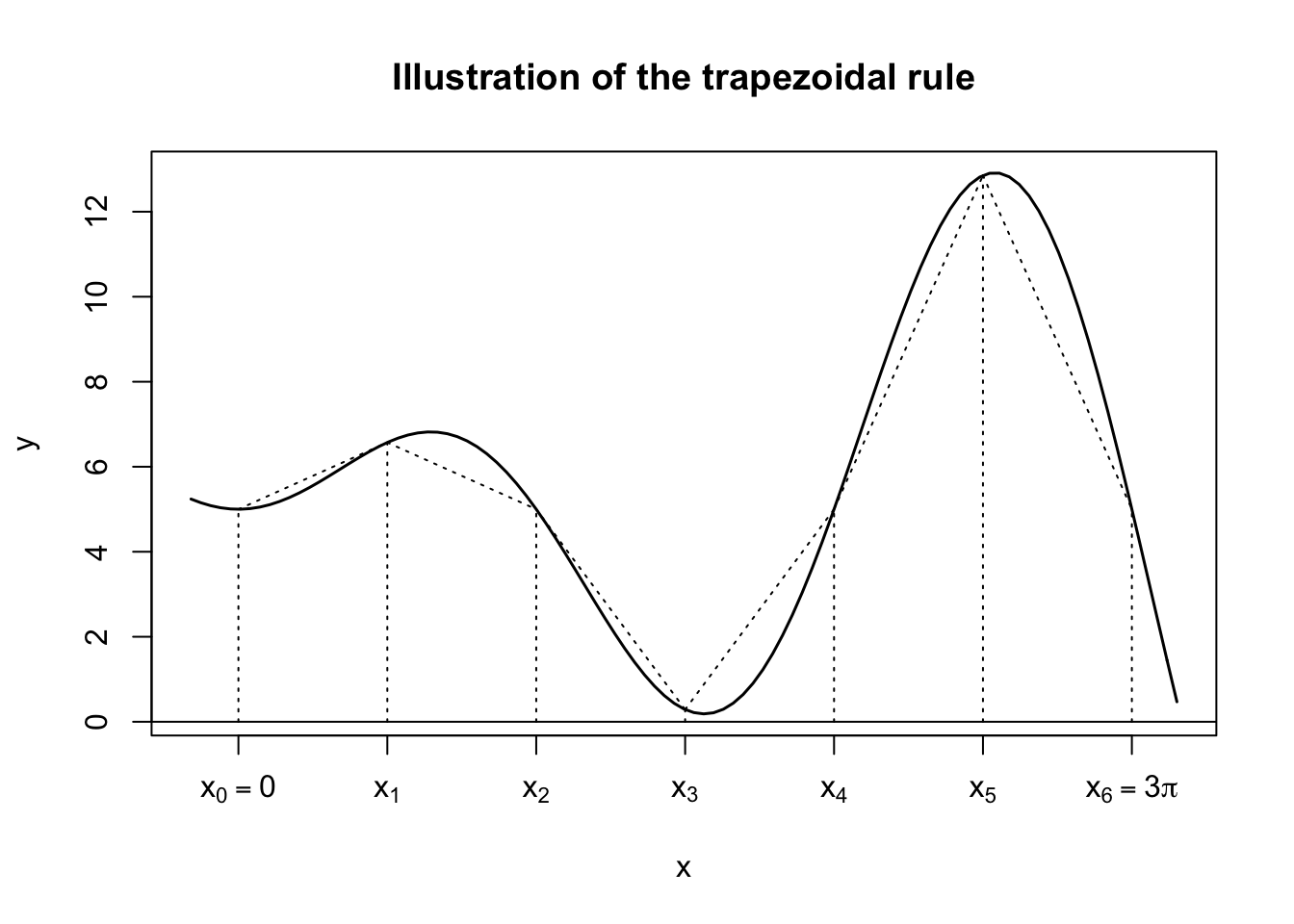These notes are based on Chapter 11 of Jones, O., Maillardet, R., & Robinson, A. (2009). Introduction to scientific programming and simulation using R. CRC Press.

# Introduction

A definite integral $$\int_a^b f(x)dx$$ is easy to compute if we can find an antiderivative $$F$$ such that $$F'(x) = f(x)$$ because $$\int_a^b f(x)dx = F(b)-F(a)$$. Unfortunately, for many functions, it is impossible to write down an antiderivative in a closed form. This is a key motivation for numerical integration, which could provide good approximations of definite integrals. For example, the cumulative distribution function (CDF) of the standard normal distribution $\Phi(z) = \int_{-\infty}^z\frac{1}{\sqrt{2\pi}}e^{-x^2/2}dx$ is commonly computed via numerical integration. R has a built-in pnorm for $$\Phi$$ and, for example, the following is a good approximation of $$\Phi(1.96)$$.

pnorm(1.96)
##  0.9750021

A general strategy in both analytical and numerical mathematics is when a function is too complex to handle, we approximate it by simple functions. However, there is a key difference between two realms. In analytical integration, an integral is defined as a limit. So, it usually does not matter whether we approximate the integrand by functions or constant values. In numerical integration, on the other hand, we always have to settle for finite granularity, so the choice of simple functions affects the performance of approximation.

In these notes, we consider linear and quadratic approximations. Specifically, the following are three methods in ascending order of sophistication:

• Trapezoidal rule
• Simpson’s rule

These are deterministic methods for numerical integration. In contrast, Monte Carlo integration is a class of stochastic methods.

A test problem is to integrate $$f(x) = x\sin(x)+5$$ over $$[0,3\pi]$$, which can be analytically computed using integration by parts. \begin{align} \int_0^{3\pi} x\sin(x)+5 dx &= \int_0^{3\pi} x\sin(x) dx + \int_0^{3\pi} 5 dx\\ &= \left( -x\cos(x)\Bigr\rvert_0^{3\pi} + \int_0^{3\pi}\cos(x)dx \right) + 15\pi\\ &= 18\pi\\ &\approx 56.5486677646 \end{align}

f <- function(x) x*sin(x)+5
integrate(f, 0, 3*pi) # R built-in function
## 56.54867 with absolute error < 8e-12

# Trapezoidal rule

Given a finite set of subintervals, the trapezoidal rule approximates the area under $$y=f(x)$$ by the finite sum of trapezoid areas, which are easy to compute. For example, using $$n=6$$ subintervals,

curve(x*sin(x)+5, xaxt="n", lwd=1.5, from=-.5, to=9.9, ylab="y")
abline(h=0)
title("Illustration of the trapezoidal rule")
x <- seq(0, 3*pi, .5*pi)
# How can we generate these expressions using a loop?
letters <- c(expression(x==0), expression(x), expression(x),
expression(x), expression(x), expression(x),
expression(x==3*pi))
axis(1, at=x, labels=letters)
from_x <- c(0, .5*pi, pi, 1.5*pi, 2*pi, 2.5*pi, 3*pi)
from_y <- rep(0,7)
to_x <- from_x
to_y <- sapply(from_x, function(x) x*sin(x)+5)
segments(from_x, from_y, to_x, to_y, lty=3)

from_x <- from_x[-length(from_x)]
from_y <- to_y[-length(to_y)]
to_x <- to_x[-1]
to_y <- to_y[-1]
segments(from_x, from_y, to_x, to_y, lty=3)and using $$n=12$$ subintervals,

curve(x*sin(x)+5, xaxt="n", lwd=1.5, from=-.5, to=9.9, ylab="y")
abline(h=0)
title("Illustration of the trapezoidal rule")
x <- seq(0, 3*pi, length.out=13)
# How can we generate these expressions using a loop?
letters <- c(expression(x==0), expression(x), expression(x),
expression(x), expression(x), expression(x),
expression(x), expression(x), expression(x),
expression(x), expression(x), expression(x),
expression(x==3*pi))
axis(1, at=x, labels=letters)
from_x <- seq(0, 3*pi, length.out=13)
from_y <- rep(0,13)
to_x <- from_x
to_y <- sapply(from_x, function(x) x*sin(x)+5)
segments(from_x, from_y, to_x, to_y, lty=3)

from_x <- from_x[-length(from_x)]
from_y <- to_y[-length(to_y)]
to_x <- to_x[-1]
to_y <- to_y[-1]
segments(from_x, from_y, to_x, to_y, lty=3)